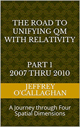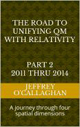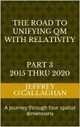# Absolute Space-time and Newton’s Bucket

In 1687 Isaac Newton proposed what has come to be called Newton’s Bucket which was an attempt to understand the nature of accelerated motion. It has been accepted for centuries that motion is relative. In other words, if two freely moving objects are each moving at constant but different velocities, neither object has a special status with respect to their motion.

 Newton’s Bucket

His experiment was meant to show that accelerated motion is different in that it is not relative. However, since velocity is relative, this raises the question of why changes in velocity (acceleration) not. The Newton’s Bucket experiment is designed to clarify the question and provide an answer.

Briefly his experiment involved hanging a bucket of water from a rope and rotating it until the rope is tightly twisted and then releasing it so it can spin. Initially, the bucket will spin but the water will remain stationary; its surface flat. As the bucket continues to spin, friction between the bucket and the water will cause the water to spin. The water’s surface will become concave. When the rope has completely untwisted, the angular momentum of the bucket and water will cause the bucket to continue to spin, the water to spin, and the rope to twist in the other direction. Finally, the twist of the rope will cause the bucket to stop spinning. However, for a time, the water will continue to spin; rope stationary, bucket stationary, and water spinning (with a concave surface).

The question Newton was trying to answer is how is rotation and its associated acceleration defined, in nature? If all motion is relative, then changes in motion can only exist as relative quantities. Could an object be said to spin, even if it were the only thing in the universe? Newton concluded that it cannot be the motion of the water relative to the bucket, because the water’s surface is most distorted when the water is spinning most rapidly at the same speed as the bucket. For him, the only explanation was that the water was spinning with respect to absolute space.

However in1905 Einstein in his Special Theory of Relativity came along and clearly showed that all motion is relative is because of the flexibility of space and time. In other words space or more correctly space-time is not absolute.  However he did attempt to explain Newton’s Bucket in terms absolute space-time as described below.

"To deny the ether is ultimately to assume that empty space has no physical qualities whatever. The fundamental facts of mechanics do not harmonize with this view. For the mechanical behavior of a corporeal system hovering freely in empty space depends not only on relative positions (distances) and relative velocities, but also on its state of rotation, which physically may be taken as a characteristic not appertaining to the system in itself. In order to be able to look upon the rotation of the system, at least formally, as something real, Newton objectiveness space. Since he classes his absolute space together with real things, for him rotation relative to an absolute space is also something real. Newton might no less well have called his absolute space what is essential is merely that besides observable objects, another thing, which is not perceptible, must be looked upon as real, to enable acceleration or rotation to be looked upon as something real"., Einstein, Albert: "Ether and the Theory of Relativity" (1920), Sidelights on Relativity (Methuen, London, 1922)

Later writing "Because it was no longer possible to speak, in any absolute sense, of simultaneous states at different locations in the aether, the aether became, as it were, four-dimensional, since there was no objective way of ordering its states by time alone."  A. Einstein (1924), "Ãœber den Ã„ther", Verhandlungen der Schweizerischen naturforschenden Gesellschaft, 105

However a more straight forward way of explaining why the surface of the water in Newton’s experiment becomes concave would be to use absolute zero or the absolute properties of energy instead of the physical properties of four dimension space-time.

Einstein told us the energy content of an object in motion is not only depended on its rest mass but also on its relative velocity with respect to an observer.

However, if one measures the energy content of two objects in motion with respect to absolute zero (a point in space where its energy is zero) and subtracts the energy component contributed to each by their rest mass; then what is left must be a function of their velocities. Therefore, their energy of motion would be relative to each other because the difference after their rest mass component has been removed will be constant  unchanging and relative when measured with respect to each other.

Acceleration is not a relative quantity because the energy content of object undergoing it does not remain constant.

For example if you know the energy component of an objects mass with respect to absolute zero and how much energy you impart to it you can calculate the magnitude of acceleration it will undergo.

In other words all acceleration can be defined with respect to absolute zero even that experienced by the water in Newton’s bucket.

For example its rotational energy with respect to energy associated with absolute zero is constantly changing because of the energy imparted to it by the rotating bucket. This would be true even for someone sitting on the edge of the bucket because radius of its circular motion is smaller near its center. Therefore its surface will become concave because, its radius increases as it approaches the sides of the bucket the energy imparted to it by its rotation will decrease.

In other words Newton was right when concluded that it cannot be the motion of the water relative to the bucket because it is moving at the same speed.  Instead one could say that it was moving relation to the energy field of the bucket.

Einstein told us field properties of space and time interact to create the mass and energy that make up our physical universe. However the equation E=mc^2 also tells us energy is equivalent to mass. Therefore, one can derive the rotational properties objects with respect to the properties of their energy components for the same reason as we can do so any of its other properties.

In other words the answer the question as to how an object can be said to spin, even if it were the only thing in the universe is that one can explain the concave surface of the water in the bucket with respect to the absolute field properties of the energy component space.

Additionally one can also understand the accelerations associated with gravity in the same terms because the curvature in space-time Einstein associated with it would correspond to an object moving though an energy field with increasing magnitude as it approached an object while following the contour of the that field.

In other words using absolute zero, (a point in space where is energy is zero) as reference point gives a universal, measurable and observable point of reference for all motion both constant and accelerated.

Latter Jeff

 The Road to Unifying QM with Relativity part 1 2007 thru 2010Ebook \$8.00Paper Back \$15.00 The Road to Unifying QM with Relativity part 2 2011 thru 2014Ebook \$8.00Paper Back \$16.00 The Road to Unifying QM with Relativity part 3 2015 thru 2020Ebook \$8.00Paper Back \$18.00